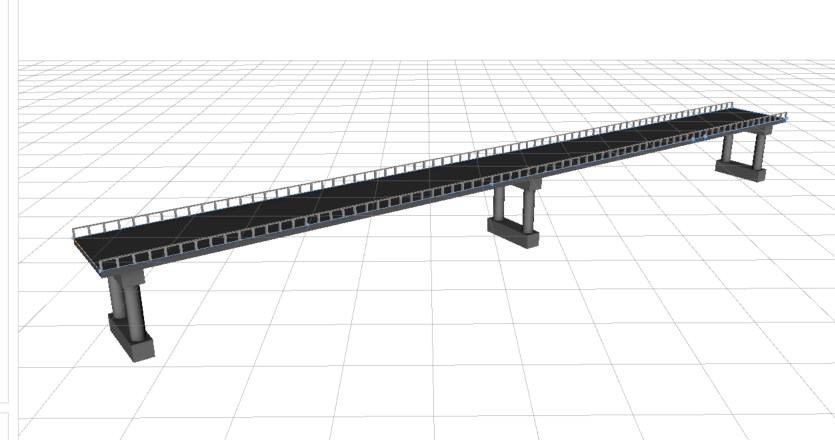# Bridge Polygon rule package

667
1
11-06-2020 06:06 AMNew Contributor III

I'm fairly new to CGA, but I want to modify the existing rule package, so the pier style would be based on attribute data. The rule packages is originally from 3D Basemaps Solution. Currently, the only options for pier styles are "Solid", "2 Pipes", "3 Pipes", which means that throughtout the bridge, the shore and river piers stay the same style. However, based on my attribute data, the shore piers can be solid, but the river piers can be "2 Pipes" and "3 Pipes". How do I modify the rule package in a way that the inner and outer piers are different?

For example, in this picture, all the piers are solid.And in this picture they are "2 Pipes".I need to combine those two styles, so that the middle pier is "2 Pipes" and the end and start piers are solid blocks.

This is the part of the code which defines pier attributes, which I have modified a little bit. I believe I have to change the split(x) part and PierType.

pierBaseWidth = bridgeWidth * ((0.8)) #double parentheses denote undeclared constant.
pierBaseLength = pierBaseWidth * Jalami_pikkus

lengthBetweenEndPiers = bridgeLength - 2 *( _Pier_Start_Length + pierBaseLength)
pierCountBetweenEndPiers =
case Pier_Count == 1:
1

else:
Pier_Count - 2

emptySpanTotal = lengthBetweenEndPiers - (pierCountBetweenEndPiers * pierBaseLength)
emptySpan = emptySpanTotal / (pierCountBetweenEndPiers + 1)

@Hidden

Piers -->
#alignScopeToAxes(y)
#t(0,10,0)
color("#808080")
PierCounts

PierCounts -->
case Pier_Count == 1:
# put 1 in the middle, but use same split pattern as Pier_Count >= 3,
# but without the repeat split operator
split(x){ _Pier_Start_Length : NIL.
| pierBaseLength: NIL.
| {{emptySpan: NIL. | pierBaseLength: PierBase} | emptySpan: NIL.}
| pierBaseLength: NIL.
| _Pier_Start_Length : NIL.}
case Pier_Count == 2:
split(x){ _Pier_Start_Length : NIL
| pierBaseLength: PierBase
| ~1: NIL
| pierBaseLength: PierBase
| _Pier_Start_Length : NIL}
case Pier_Count >= 3:
split(x){ _Pier_Start_Length : NIL.
| pierBaseLength: PierBase
| {{emptySpan: NIL. | pierBaseLength: PierBase}* | emptySpan: NIL.}
| pierBaseLength: PierBase
| _Pier_Start_Length : NIL.}
else:
NIL

#onePierEmptySpan = (bridgeLength - (_Pier_Start_Length + pierBaseLength)) / 2 #(_Pier_Start_Length + pierBaseLength)
slopedPierHypotenuse =
emptySpan + pierBaseLength

yDiffPerIndex =
case pier_Slope_Flip:
yDiff * reverseIndex
else:
yDiff * pierIndex

# 1,3,5,7, ... --> 0,1,2,3, ...
zeroBaseOddIndex(oddValue) = (oddValue - 1) / 2
reverseIndex = Pier_Count - pierIndex - 1

@Hidden
attr pierIndex = 0

PierBase -->
case Pier_Count == 1:
set(pierIndex, 1) # not 0.
extrude(world.up.flatTop,-( _Pier_Height +yDiffPerIndex))
PierScale
else:
set(pierIndex, zeroBaseOddIndex(split.index))
extrude(world.up.flatTop,-( _Pier_Height +yDiffPerIndex))
PierScale

PierScale -->
s('1,'1, pierBaseWidth)
center(z)
PierVerticalSplits

PierVerticalSplits -->
split(y){pierFootingHeight: X. | ~1: PierMiddle | pierFootingHeight: X.}

PierMiddle -->
s('0.7,'1,'0.9)
center(xz)
PierDimensions

pierFootingHeight = lesserOf( _Pier_Height * ((0.25)), pierFootingHeightMax)

@Hidden
attr footShort = 0
@Hidden
attr footLong = 0
@Hidden
attr footMiddleHeight = 0

PierDimensions -->
alignScopeToAxes(y)
set(footMiddleHeight, scope.sy)
set(footShort, scope.sx)
set(footLong, scope.sz)
PierType

PierType -->
case Pier_Type == "2 Pipes":
split(z){footShort: Pipe | ~1: NIL | footShort: Pipe}
case Pier_Type == "3 Pipes":
split(z){footShort: Pipe | ~1: NIL
| footShort: Pipe | ~1: NIL | footShort: Pipe}
else:
X.

Pipe -->
primitiveCylinder()

Tags (4)
1 Solution

Accepted SolutionsbyEsri Regular Contributor

Check out this simple example that colors the inner piers red and the outer piers green.

``attr Inner_Pier_Type = "2 Pipes"attr Outer_Pier_Type = "Solid"@Hiddenattr Pier_Type = "Solid"const _Pier_Start_Length = 10const pierBaseLength = 20const emptySpan = 40PiersCounts -->	split(x) { ~_Pier_Start_Length: NIL			 | pierBaseLength: set(Pier_Type, Outer_Pier_Type) PierBase			 | { ~emptySpan: NIL			   | pierBaseLength: set(Pier_Type, Inner_Pier_Type) PierBase }*			 | ~emptySpan: NIL			 | pierBaseLength: set(Pier_Type, Outer_Pier_Type) PierBase			 | ~_Pier_Start_Length: NIL }PierBase -->	case Pier_Type=="2 Pipes":		color(1,0,0)	else:		color(0,1,0)‍‍‍‍‍‍‍‍‍‍‍‍‍‍‍‍‍‍‍‍‍‍‍‍‍‍‍‍‍‍‍‍‍‍‍‍‍‍‍‍‍‍‍‍‍‍‍‍‍‍‍‍‍‍‍‍‍‍‍‍‍‍‍‍‍‍‍‍‍‍‍‍‍‍‍‍‍‍‍‍‍‍‍‍‍‍‍‍‍‍‍‍‍‍‍``Replace the split in your PiersCounts rule with the split in the example above.  Add attrs for the inner and outer pier styles.  In the split, set these attrs depending on whether the part is an inner part or an outer part.by``attr Inner_Pier_Type = "2 Pipes"attr Outer_Pier_Type = "Solid"@Hiddenattr Pier_Type = "Solid"const _Pier_Start_Length = 10const pierBaseLength = 20const emptySpan = 40PiersCounts -->	split(x) { ~_Pier_Start_Length: NIL			 | pierBaseLength: set(Pier_Type, Outer_Pier_Type) PierBase			 | { ~emptySpan: NIL			   | pierBaseLength: set(Pier_Type, Inner_Pier_Type) PierBase }*			 | ~emptySpan: NIL			 | pierBaseLength: set(Pier_Type, Outer_Pier_Type) PierBase			 | ~_Pier_Start_Length: NIL }PierBase -->	case Pier_Type=="2 Pipes":		color(1,0,0)	else:		color(0,1,0)‍‍‍‍‍‍‍‍‍‍‍‍‍‍‍‍‍‍‍‍‍‍‍‍‍‍‍‍‍‍‍‍‍‍‍‍‍‍‍‍‍‍‍‍‍‍‍‍‍‍‍‍‍‍‍‍‍‍‍‍‍‍‍‍‍‍‍‍‍‍‍‍‍‍‍‍‍‍‍‍‍‍‍‍‍‍‍‍‍‍‍‍‍‍‍``